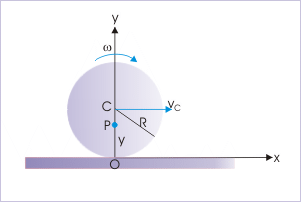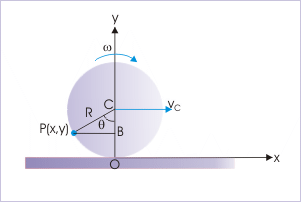# 19.3 Rolling as pure rotation (exercise)

 Page 1 / 2
Solving problems is an essential part of the understanding process.

Questions and their answers are presented here in the module text format as if it were an extension of the theoretical treatment of the topic. The idea is to provide a verbose explanation of the solution, detailing the application of theory. Solution presented here, therefore, is treated as the part of the understanding process – not merely a Q/A session. The emphasis is to enforce ideas and concepts, which can not be completely absorbed unless they are put to real time situation.

## Representative problems and their solutions

We discuss problems, whose analysis is suited to the technique of treating rolling motion as pure rotation. For this reason, questions are categorized in terms of the characterizing features pertaining to the questions :

• Positions on the rolling body with a specified velocity
• Velocity of a particle situated at a specified position
• Distance covered by a particle in rolling
• Kinetic energy of rolling

## Position on the rolling body with specified velocity

Example 1

Problem : At an instant, the contact point of a rolling disk of radius “R” coincides with the origin of the coordinate system. If the disk rolls with constant angular velocity, “ω”, along a straight line, then find the position of a particle on the vertical diameter, whose velocity is 1/ 2 of the velocity with which the disk rolls.

Solution : Here, the particle on the vertical diameter moves with a velocity, which is 1/ 2 of that of the velocity of the center of mass. Now, velocity of center of mass is :

$\begin{array}{l}{v}_{C}=\omega R\end{array}$

Let the particle be at a distance “y” from the point of contact on the vertical diameter. Then, velocity of the particle is :Velocity of a particle on the vertical diameter

$\begin{array}{l}v=\omega r=\omega y\end{array}$

According to question,

$\begin{array}{l}v=\frac{{v}_{C}}{2}\end{array}$

Putting values,

$\begin{array}{l}⇒\omega y=\frac{{v}_{C}}{2}=\frac{\omega R}{2}\end{array}$

$\begin{array}{l}⇒y=\frac{R}{2}\end{array}$

This result is expected from the nature of relation “ $v=\omega r$ ”. It is a linear relation for vertical distance. The velocity varies linearly with the vertical distance.

Example 2

Problem : At an instant, the contact point of a rolling disk of radius “R” coincides with the origin of the coordinate system. If the disk rolls with constant angular velocity, “ω”, along a straight line, then find the position of a particle on the rim of the disk, whose speed is same as the speed with which the disk rolls.

Solution : Here, the particle on the rim of the disk moves with the same velocity as that of the velocity of the center of mass. Now, velocity of center of mass is :

$\begin{array}{l}{v}_{C}=\omega R\end{array}$

Let the particle be at P(x,y) as shown in the figure. Then, velocity of the particle is :Velocity of a particle on the rim of the disk

$\begin{array}{l}v=2{v}_{C}\mathrm{sin}\left(\frac{\theta }{2}\right)=2\omega R\mathrm{sin}\left(\frac{\theta }{2}\right)\end{array}$

According to question,

$\begin{array}{l}v={v}_{C}\end{array}$

Putting values,

$\begin{array}{l}2{v}_{C}\mathrm{sin}\left(\frac{\theta }{2}\right)=2\omega R\mathrm{sin}\left(\frac{\theta }{2}\right)=\omega R\end{array}$

$\begin{array}{l}⇒\mathrm{sin}\left(\frac{\theta }{2}\right)=\frac{1}{2}=\mathrm{sin}\mathrm{30}°\\ ⇒\frac{\theta }{2}=\mathrm{30}°\\ ⇒\theta =\mathrm{60}°\end{array}$

$\begin{array}{l}⇒x=-R\mathrm{sin}\mathrm{60}°=-\frac{\surd 3R}{2}\end{array}$

$\begin{array}{l}⇒y=R\mathrm{cos}\mathrm{60}°=\frac{R}{2}\end{array}$

Since there are two such points on the rim on either side of the vertical line, the coordinates of the positions of the particles, having same speed as that of center of mass are :

$\begin{array}{l}-\frac{\surd 3R}{2},\phantom{\rule{2pt}{0ex}}\frac{R}{2}\phantom{\rule{4pt}{0ex}}\mathrm{and}\phantom{\rule{4pt}{0ex}}\frac{\surd 3R}{2},\phantom{\rule{2pt}{0ex}}\frac{R}{2}\end{array}$

## Velocity of a particle situated at a specified position

Example 3

#### Questions & Answers

What are the system of units
Jonah Reply
A stone propelled from a catapult with a speed of 50ms-1 attains a height of 100m. Calculate the time of flight, calculate the angle of projection, calculate the range attained
Samson Reply
58asagravitasnal firce
Amar
water boil at 100 and why
isaac Reply
what is upper limit of speed
Riya Reply
what temperature is 0 k
Riya
0k is the lower limit of the themordynamic scale which is equalt to -273 In celcius scale
Mustapha
How MKS system is the subset of SI system?
Clash Reply
which colour has the shortest wavelength in the white light spectrum
Mustapha Reply
how do we add
Jennifer Reply
if x=a-b, a=5.8cm b=3.22 cm find percentage error in x
Abhyanshu Reply
x=5.8-3.22 x=2.58
sajjad
what is the definition of resolution of forces
Atinuke Reply
what is energy?
James Reply
Ability of doing work is called energy energy neither be create nor destryoed but change in one form to an other form
Abdul
motion
Mustapha
highlights of atomic physics
Benjamin
can anyone tell who founded equations of motion !?
Ztechy Reply
n=a+b/T² find the linear express
Donsmart Reply
أوك
عباس
Quiklyyy
Sultan Reply
Moment of inertia of a bar in terms of perpendicular axis theorem
Sultan Reply
How should i know when to add/subtract the velocities and when to use the Pythagoras theorem?
Yara Reply

### Read also:

#### Get the best Physics for k-12 course in your pocket!

Source:  OpenStax, Physics for k-12. OpenStax CNX. Sep 07, 2009 Download for free at http://cnx.org/content/col10322/1.175
Google Play and the Google Play logo are trademarks of Google Inc.

Notification Switch

Would you like to follow the 'Physics for k-12' conversation and receive update notifications?ByByByBy